Miscellaneous

Chapter 3 Class 11 Trigonometric Functions
Serial order wise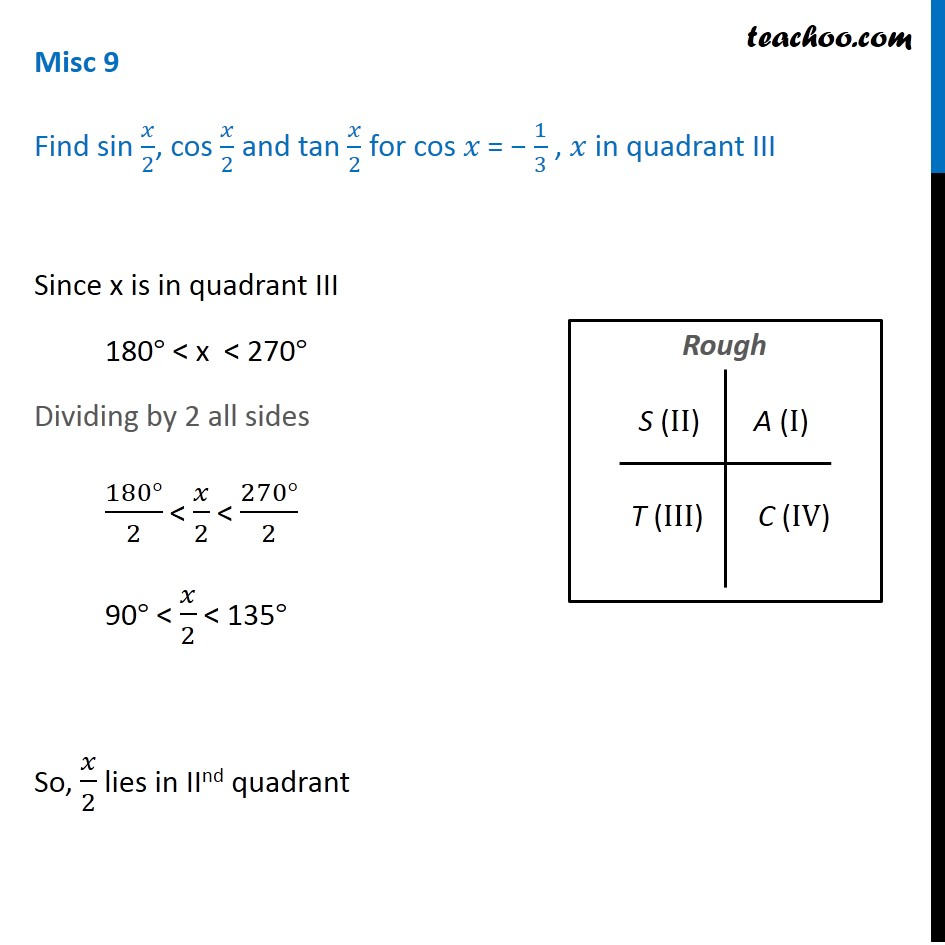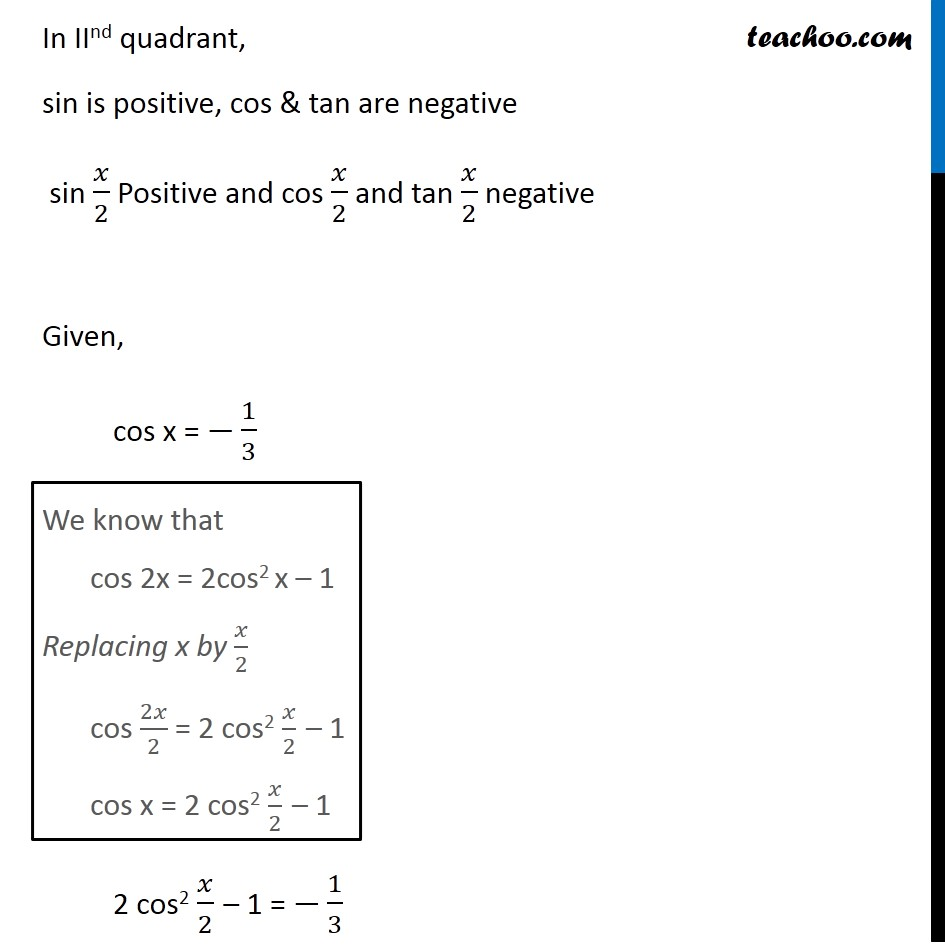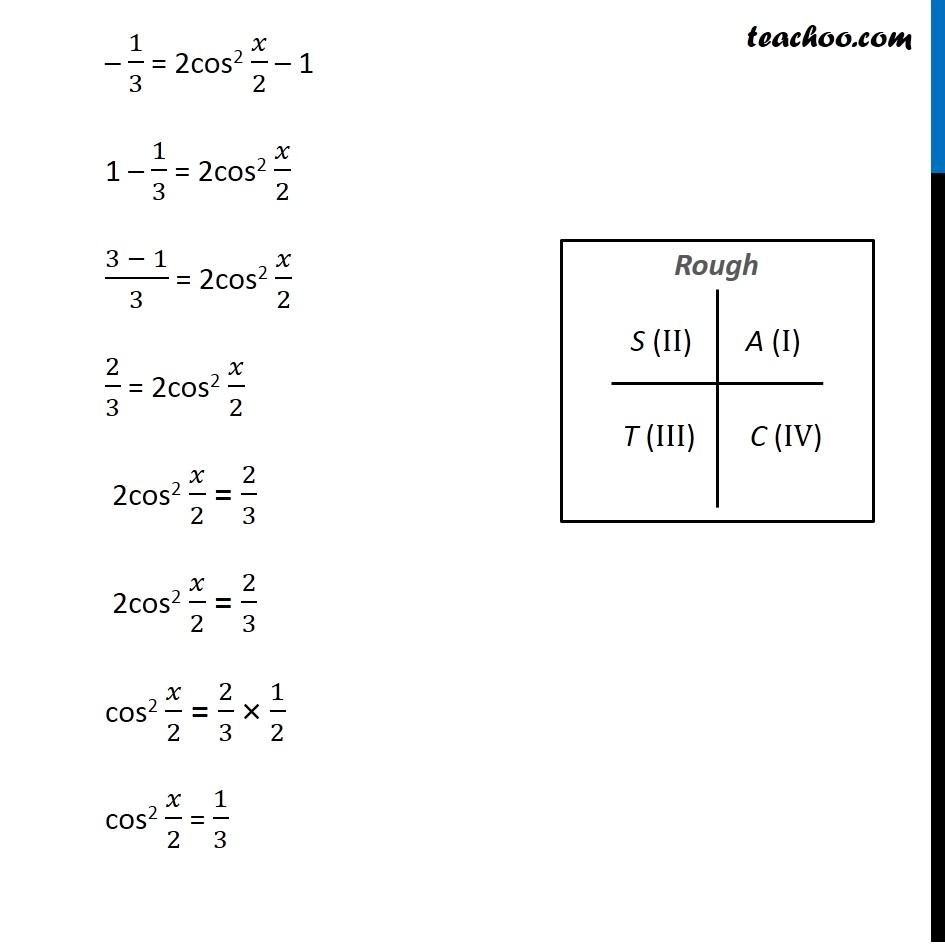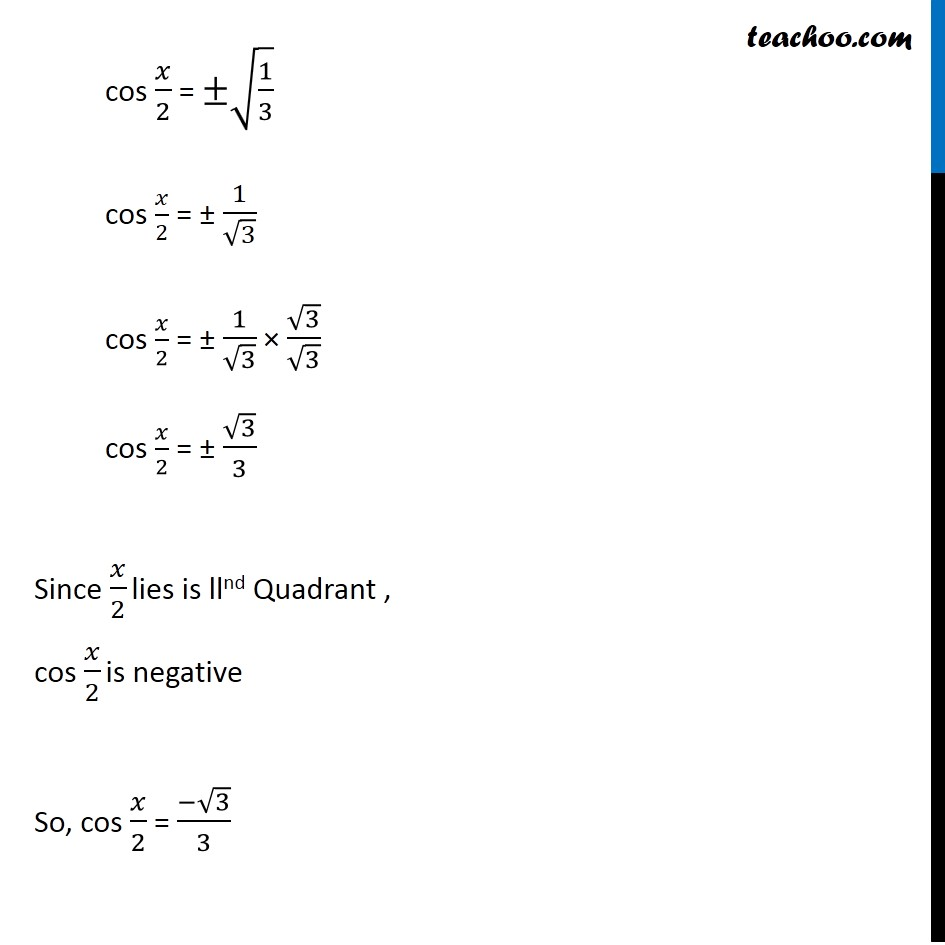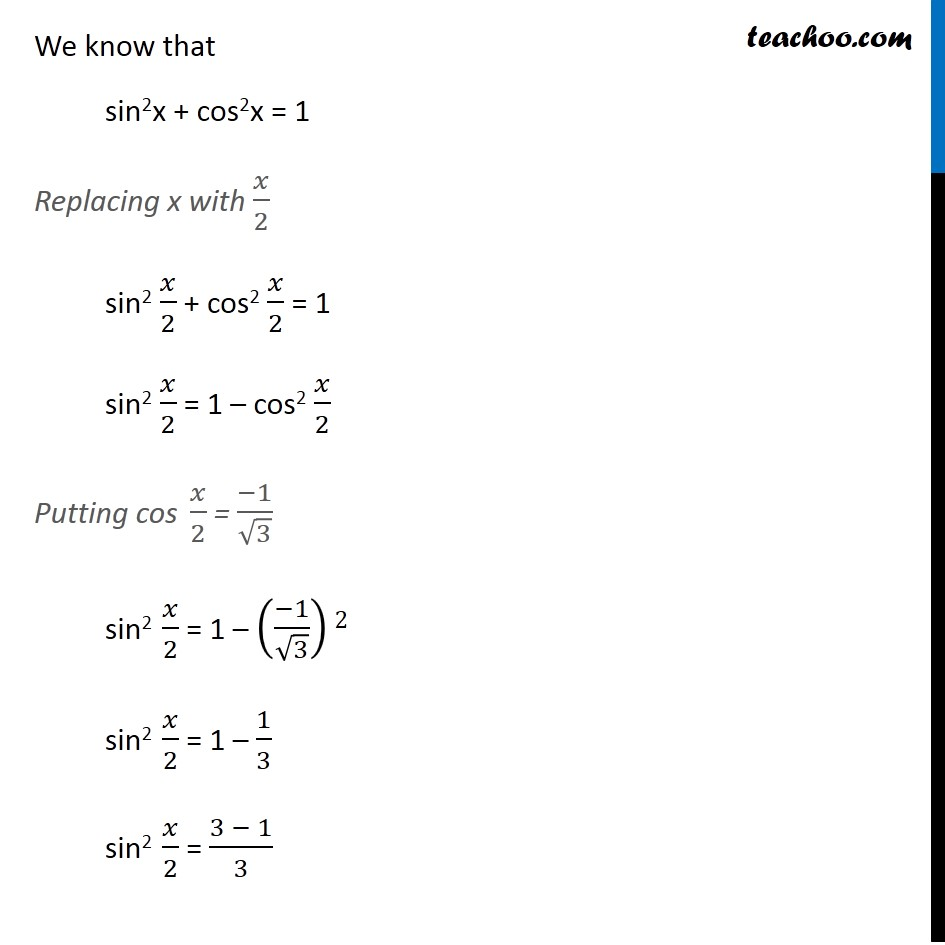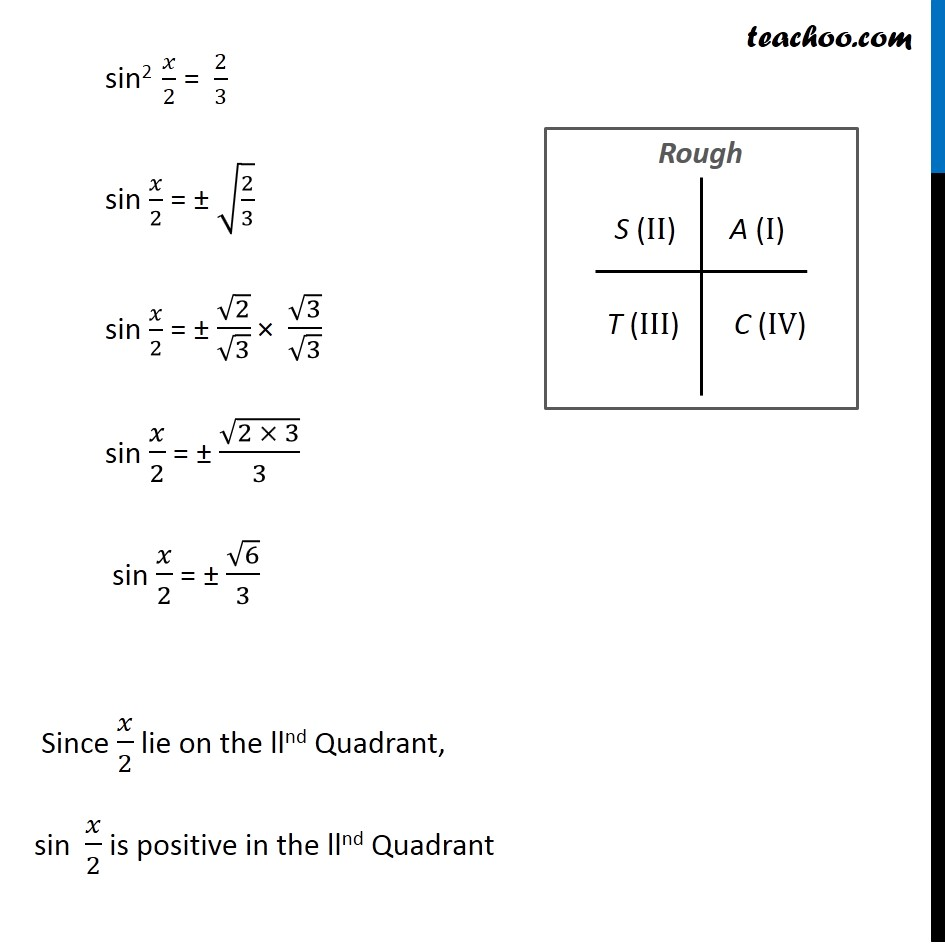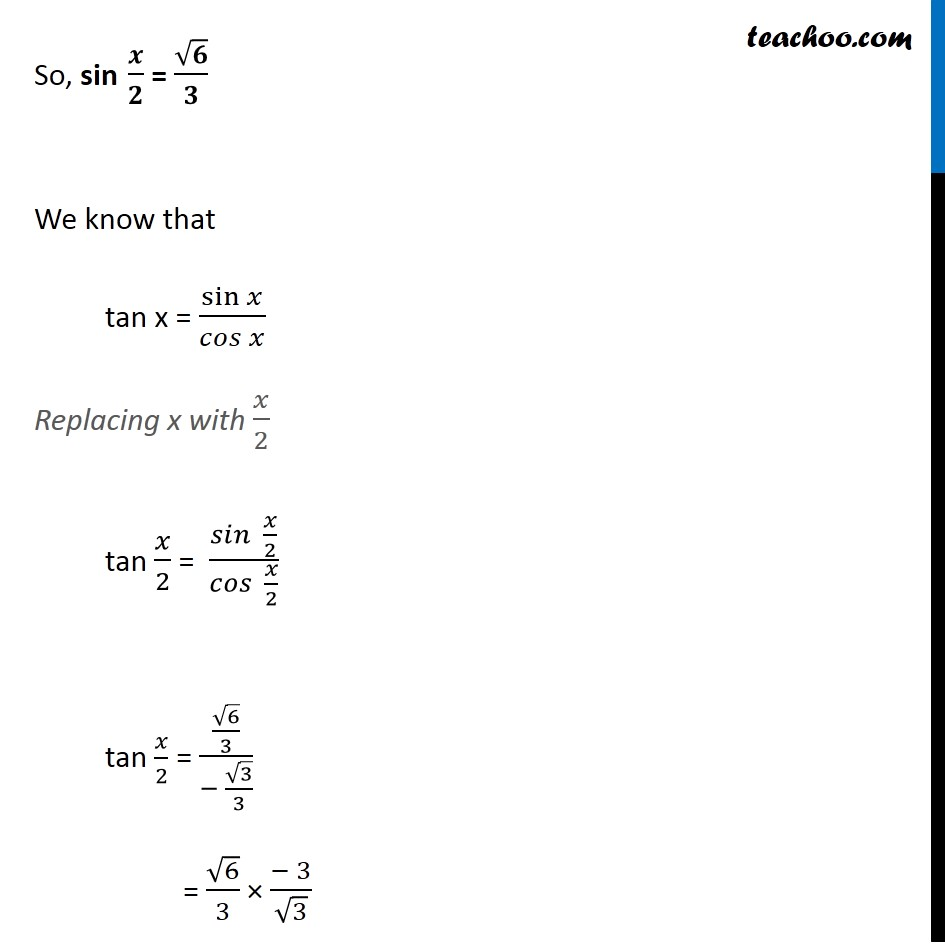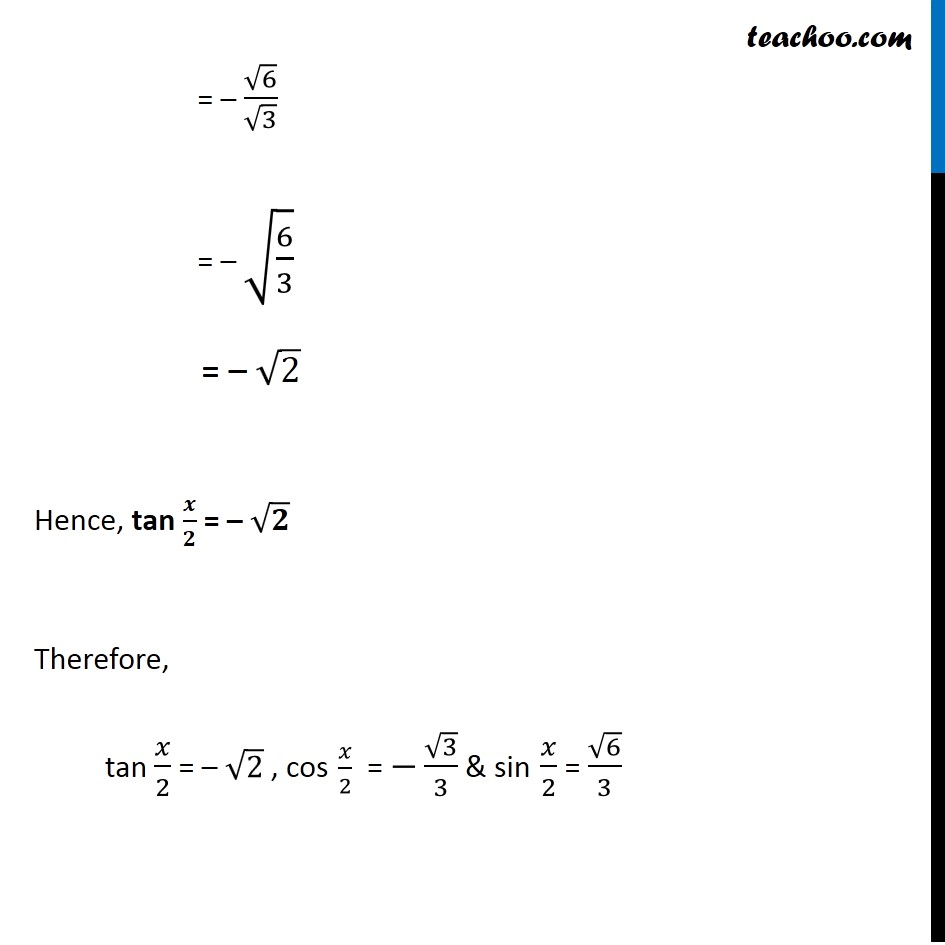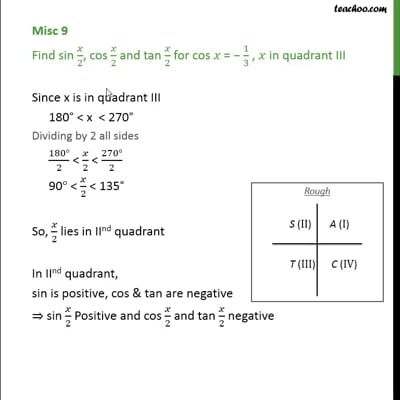This video is only available for Teachoo black users

Solve all your doubts with Teachoo Black (new monthly pack available now!)

### Transcript

Misc 9 Find sin /2, cos /2 and tan /2 for cos = 1/3 , in quadrant III Since x is in quadrant III 180 < x < 270 Dividing by 2 all sides (180 )/2 < /2 < (270 )/2 90 < /2 < 135 So, /2 lies in IInd quadrant In IInd quadrant, sin is positive, cos & tan are negative sin /2 Positive and cos /2 and tan /2 negative Given, cos x = 1/3 cos x = 2 cos2 /2 1 1/3 = 2cos2 /2 1 1 1/3 = 2cos2 /2 (3 1)/3 = 2cos2 /2 2/3 = 2cos2 /2 2cos2 /2 = 2/3 cos2 /2 = 2/3 1/2 cos2 /2 = 1/3 cos /2 = (1/3) = 1/ 3 Since /2 lie is llnd Quadrant , cos /2 is negative So cos /2 = 1/ 3 = 1/ 3 3/ 3 = 3/3 We know that sin2x + cos2x = 1 Replacing x with /2 sin2 /2 + cos2 /2 = 1 sin2 /2 = 1 cos2 /2 Putting cos /2 = 1/ 3 sin2 /2 = 1 (" " 1/ 3)2 sin2 /2 = 1 1/3 sin2 /2 = (3 1)/3 sin2 /2 = 2/3 sin /2 = (2/3) = 2/ 3 = 2/ 3 3/ 3 = (2 3)/3 = 6/3 Since /2 lie on the llnd Quadrant , sin /2 is positive in the llnd Quadrant So sin /2 = 6/3 We know that tan x = sin / Replacing x with /2 tan /2 = /2 / /2 = ( 6/3)/( 3/3) = 6/3 ( 3)/ 3 = 6/ 3 = (6/3) = 2 Hence, tan /2 = 2 Hence, tan /2 = 2 , cos /2 = 3/3 & sin /2 = 6/3